{{announcement.body}}
{{announcement.title}}

# Introduction to Xamarin.Forms for Android Developers (Part 3)

DZone 's Guide to

# Introduction to Xamarin.Forms for Android Developers (Part 3)

### This tutorial teaches us about layouts, classes in Xamarin.Forms for arranging visual elements in your app's user interface.

· Mobile Zone ·
Free Resource

Comment (0)

Save
{{ articles.views | formatCount}} Views

## Introduction

Layouts are classes that allow for arranging visual elements (such as controls) in the user interface (UI), and Xamarin.Forms provides many of them. In this article, we are going to discover

## Creating Layouts in Xamarin.Forms

Xamarin.Forms allows us to create layouts in two ways: XAML and C# code. We are going to discover each layout in both ways. To simplify, we are going to create a new application named LayoutsApp and suppose our UI has two controls: one Label and one Button. Controls in Xamarin.Forms will be discovered in more detail in the next article.

### The StackLayout

StackLayout allows us to place controls near each other horizontally or vertically. We can create this layout that contains our two controls by using XAML (in the MainPage.xaml file) as follows:

``````<StackLayout Orientation="Vertical" Margin="5">
<Label Text="I am a Lablel" Margin="5"/>
<Button Text="I am a Button" Margin="5"/>
</StackLayout>``````

We can create the StackLayout by using C# code (in the MainPage.xaml.cs) as follows:

``````var layout = new StackLayout();

layout.Orientation = StackOrientation.Vertical;

layout.Margin = 5;

var myLabel = new Label
{
Text = "I am a Label",
Margin = 5
};

var myButton = new Button
{
Text = "I am a Button",
Margin = 5
};

Content = layout;``````

Result: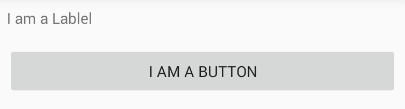Or using XAML:

``````<StackLayout Orientation="Horizontal" Margin="5">
<Label Text="I am a Lablel" Margin="5"/>
<Button Text="I am a Button" Margin="5"/>
</StackLayout>``````

and C# code:

``````var layout = new StackLayout();

layout.Orientation = StackOrientation.Horizontal;

layout.Margin = 5;

var myLabel = new Label
{
Text = "I am a Label",
Margin = 5
};

var myButton = new Button
{
Text = "I am a Button",
Margin = 5;
};

Content = layout;``````

Result: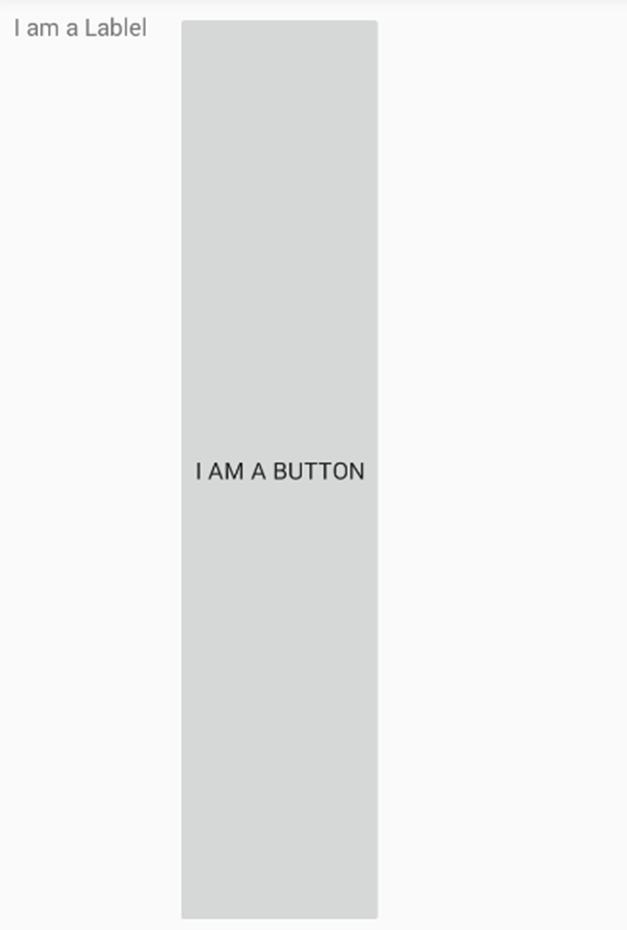### The Grid

The Grid allows us to organize controls within rows and columns.

Using XAML in the MainPage.xaml file:

``````<!-- one row, two columns-->
<Grid>
<!-- one row -->
<Grid.RowDefinitions>
<RowDefinition />
</Grid.RowDefinitions>
<!-- two columns -->
<Grid.ColumnDefinitions>
<ColumnDefinition />
<ColumnDefinition />
</Grid.ColumnDefinitions>
<!-- Grid.Row="0" and Grid.Column="0" can be omitted -->
<Label Text="I am a Lablel" Margin="5"/>
<Button Grid.Column="1" Text="I am a Button" Margin="5"/>
</Grid>``````

Using C# code in the MainPage.xaml.cs:

``````// create a new grid

var grid = new Grid();

// define one row

//define two columns

var myLabel = new Label

{

Text = "I am a Label",

Margin = 5,

};

var myButton = new Button

{

Text = "I am a Button",

Margin = 5

};

// Grid.Row = 0 Grid.Column = 0

// Grid.Row = 0 Grid.Column = 1

Content = grid;``````

Result: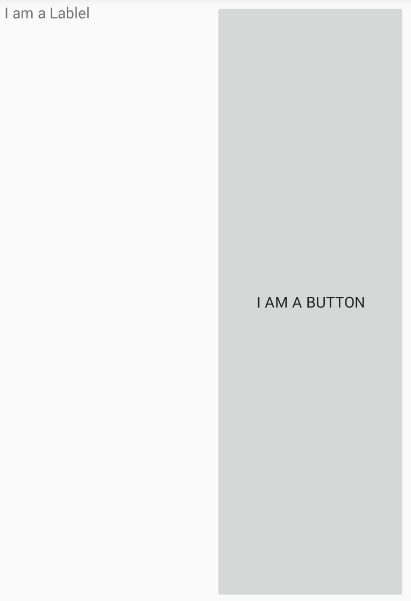Or using XAML:

``````<!-- two rows, one column-->
<Grid>
<!-- two rows -->
<Grid.RowDefinitions>
<RowDefinition />
<RowDefinition />
</Grid.RowDefinitions>
<!-- one column -->
<Grid.ColumnDefinitions>
<ColumnDefinition />
</Grid.ColumnDefinitions>
<!-- Grid.Row="0" and Grid.Column="0" can be omitted -->
<Label Text="I am a Lablel" Margin="5"/>
<Button Grid.Row="1" Text="I am a Button" Margin="5"/>
</Grid>``````

and using C# code:

``````// create a new grid

var grid = new Grid();

// define two rows

//define one column

var myLabel = new Label

{

Text = "I am a Label",

Margin = 5,

};

var myButton = new Button

{

Text = "I am a Button",

Margin = 5

};

// Grid.Row = 0 Grid.Column = 0

// Grid.Row = 1 Grid.Column = 0

Content = grid;

//InitializeComponent();``````

Result: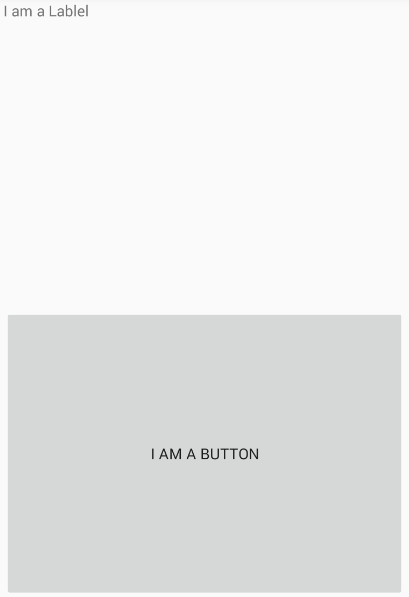### The AbsoluteLayout

The AbsoluteLayout allows we to specify where exactly on the screen we want the controls to appear, as well as their size and bounds.

Using XAML in the MainPage.xaml file:

``````<AbsoluteLayout>

<!--x:0 y:0 Width:0.25 Height:0.25 -->

<Label Text="I am a Lablel" Margin="5" BackgroundColor="Red"
AbsoluteLayout.LayoutBounds="0, 0, 0.25, 0.25"
AbsoluteLayout.LayoutFlags="All"/>

<!--x:0.20 y:0.20 Width:0.25 Height:0.25 -->

<Button Text="I am a Button" Margin="5"
AbsoluteLayout.LayoutBounds="0.20, 0.20, 0.25, 0.25"
AbsoluteLayout.LayoutFlags="All" />

</AbsoluteLayout>``````

Using C# code in the MainPage.xaml.cs:

``````//create a new layout

var layout = new AbsoluteLayout();

var myLabel = new Label

{

Text = "I am a Label",

Margin = 5,

BackgroundColor = Color.Red

};

var myButton = new Button

{

Text = "I am a Button",

Margin = 5

};

AbsoluteLayout.SetLayoutBounds(myLabel, new Rectangle(0, 0, 0.25, 0.25));

AbsoluteLayout.SetLayoutFlags(myLabel, AbsoluteLayoutFlags.All);

AbsoluteLayout.SetLayoutBounds(myButton, new Rectangle(0.20, 0.20, 0.25, 0.25));

AbsoluteLayout.SetLayoutFlags(myButton, AbsoluteLayoutFlags.All);

Content = layout;``````

Result: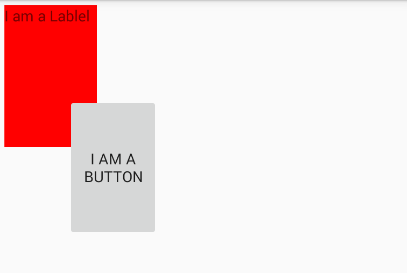### The RelativeLayout

The RelativeLayout provides a way to specify the location of child controls relative either to each other or to the parent control.

Using XAML in the MainPage.xaml file:

``````<RelativeLayout>
<Label Text="I am a Lablel" Margin="5" BackgroundColor="Blue"
x:Name="myLabel" RelativeLayout.XConstraint="{ConstraintExpression ,
,,}"
RelativeLayout.YConstraint="{ConstraintExpression ,
,Factor=.15,}"
RelativeLayout.WidthConstraint="{ConstraintExpression ,
,,}"
RelativeLayout.HeightConstraint="{ConstraintExpression ,
,Factor=.8,}"/>
<Button Text="I am a Button" Margin="5" BackgroundColor="Red"
RelativeLayout.YConstraint="{ConstraintExpression , ,
,,}"
RelativeLayout.XConstraint="{ConstraintExpression ,,
,,}"
RelativeLayout.WidthConstraint="{ConstraintExpression ,
,Factor=.5,}"
RelativeLayout.HeightConstraint="{ConstraintExpression  ,
,Factor=.5,}"/>
</RelativeLayout>``````

Using C# code in the MainPage.xaml.cs:

``````//create a new layout

var layout = new RelativeLayout();

var myLabel = new Label

{

Text = "I am a Label",

Margin = 5,

BackgroundColor = Color.Blue

};

var myButton = new Button

{

Text = "I am a Button",

Margin = 5,

BackgroundColor = Color.Red

};

return parent.X;

}), Constraint.RelativeToParent((parent) => {

return parent.Y * .15;

}), Constraint.RelativeToParent((parent) => {

return parent.Width;

}), Constraint.RelativeToParent((parent) => {

return parent.Height * .8;

}));

layout.Children.Add(myButton, Constraint.RelativeToView(myLabel, (Parent, sibling) => {

return sibling.X + 20;

}), Constraint.RelativeToView(myLabel, (parent, sibling) => {

return sibling.Y + 20;

}), Constraint.RelativeToParent((parent) => {

return parent.Width * .5;

}), Constraint.RelativeToParent((parent) => {

return parent.Height * .5;

}));

Content = layout;``````

Result: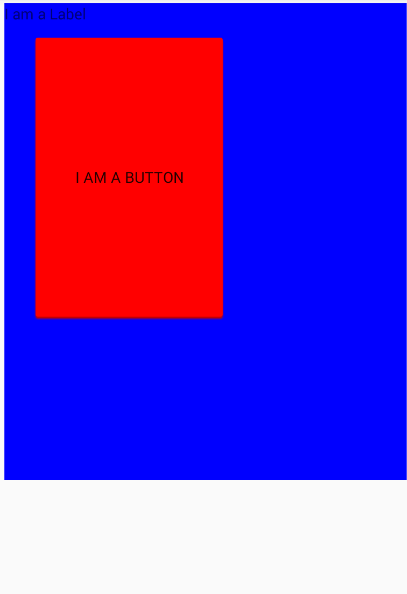### The ScrollView

The ScrollView allows we to present content that cannot fit on one screen and therefore should be scrolled.

Using XAML in the MainPage.xaml file:

``````<ScrollView>
<Label Text="I am a Lablel" Margin="5"
BackgroundColor="Red" HeightRequest="600"
WidthRequest="150"/>
</ScrollView>``````

Using C# code in the MainPage.xaml.cs:

``````  var scroll = new ScrollView();

var myLabel = new Label

{

Text = "I am a Label",

Margin = 5,

BackgroundColor = Color.Red,

,

WidthRequest = 150

};

scroll.Content = myLabel;

Content = scroll;``````

Result: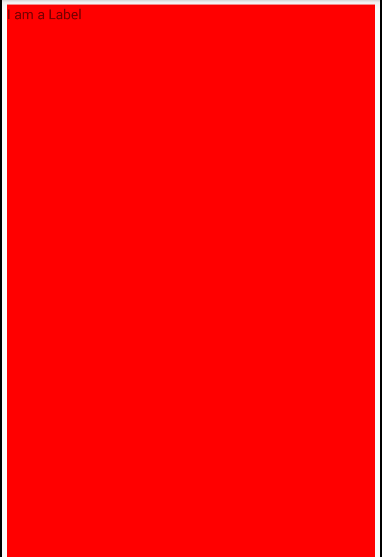We can try to scroll up or down by using the right scroll bar.

### The Frame

The Frame provides an option to draw a colored border around the controls it contains, and optionally add extra space between the Frame’s bounds and the controls.

Using XAML in the MainPage.xaml file:

``````<Frame OutlineColor="Red" CornerRadius="10" HasShadow="True" Margin="20" >

<Label Text="I am a Lablel" Margin="5" BackgroundColor="Red"
HorizontalOptions="Center" VerticalOptions="Center"/>

</Frame>``````

Using C# code in the MainPage.xaml.cs:

`````` var frame = new Frame();
var myLabel = new Label
{
Text = "I am a Label",
Margin = 5,
BackgroundColor = Color.Red,
HorizontalOptions= LayoutOptions.Center,
VerticalOptions = LayoutOptions.Center
};
frame.Content = myLabel;
frame.OutlineColor = Color.Red;
frame.Margin = 20;

Content = frame;``````

Result: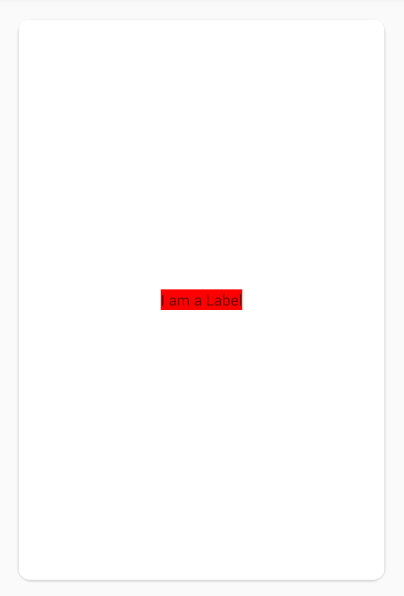We can not see the outline color in the figure above. This problem is outside the scope of this article but you can find solutions here.

### The ContentView

The ContentView contains hierarchies of visual elements and can be used to create custom controls in XAML.

Using XAML in the MainPage.xaml file:

``````<ContentView BackgroundColor="Aqua" Margin="10" Padding="25">

<Label Text="I am a Label" HorizontalOptions="Center"
VerticalOptions="Center" BackgroundColor="Red"/>

</ContentView>``````

Using C# code in the MainPage.xaml.cs

``````var contentView = new ContentView();

var myLabel = new Label

{

Text = "I am a Label",

BackgroundColor = Color.Red,

HorizontalOptions = LayoutOptions.Center,

VerticalOptions = LayoutOptions.Center

};

contentView.Content = myLabel;

contentView.Margin = 10;

contentView.BackgroundColor = Color.Aqua;

Content = contentView;``````

Result: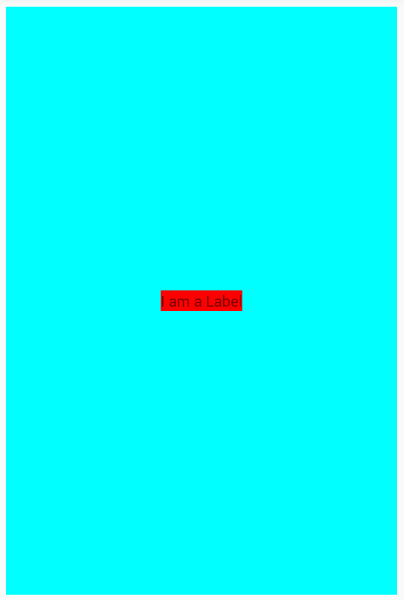## Conclusion

In this article, I introduced how to create basic layouts in two ways: XAML and C# code. You can discover more about layouts in Xamarin.Forms by referring to the Microsoft docs. In the next article, we will learn about controls in Xamarin.Forms.

Topics:
mobile ,mobile app development ,tutorial ,xamarin ,xamarin.forms ,android ,layouts ,ui

Comment (0)

Save
{{ articles.views | formatCount}} Views

Opinions expressed by DZone contributors are their own.